Question

# If a Prisoner's Dilemma game is repeated daily, such that two rival stores choose a price...

If a Prisoner's Dilemma game is repeated daily, such that two rival stores choose a price simultaneously each morning for an extended number of days, which outcome can happen?
 The Nash equilibrium will continue to be played only until one firm engages a trigger strategy against the other. There will more likely be cooperation to achieve an outcome different from the Nash equilibrium that is better for both firms. The Nash equilibrium will continue to be played throughout the game. An outcome different from the Nash equilibrium would occur; however, only one firm will be better off.

As this is an infinite game, they will not be playing on the Nash equilibrium because the game is a Prisoners dilemma and Nash equilibrium will hurt both the players, the answer is "B" There will more likely be cooperation to achieve an outcome different from the Nash equilibrium that is better for both firms.

#### Earn Coins

Coins can be redeemed for fabulous gifts.

Similar Homework Help Questions
• ### n an infinitely repeated prisoner’s dilemma (such as a repeated price war): Group of answer choices...

n an infinitely repeated prisoner’s dilemma (such as a repeated price war): Group of answer choices repeated defection is the only equilibrium. there are two different equilibria: repeated defection and repeated cooperation. repeated cooperation is the only equilibrium. each player cooperates in the early stages, but defects near the end of the game. there is no stable equilibrium strategy for either player.

• ### The dilemma in a prisoner's dilemma is that: Multiple Choice only one player has a dominant...

The dilemma in a prisoner's dilemma is that: Multiple Choice only one player has a dominant strategy, but the other player is uncertain about what to do. the players would be better off if they both played a dominated strategy. the players may be trapped in a game they don’t know how to play. the outcome is random, so players are uncertain about which strategy to play.

• ### 3. (Level A) Suppose the following Prisoner's Dilemma is repeated infinitely: 112 C D C 2,...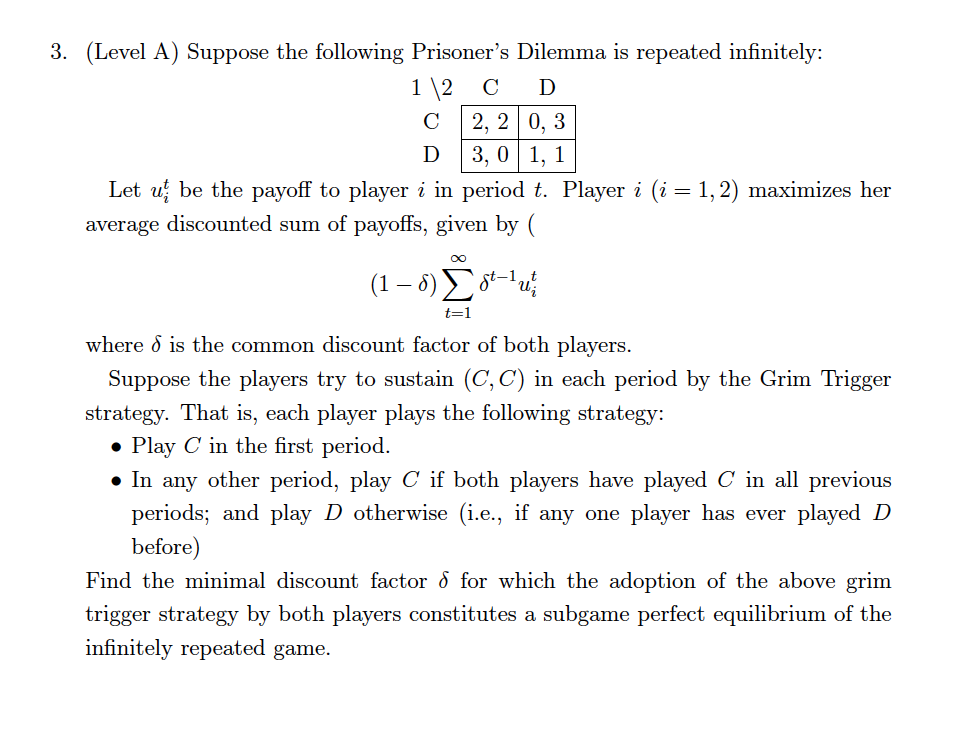3. (Level A) Suppose the following Prisoner's Dilemma is repeated infinitely: 112 C D C 2, 2 0, 3 D 3,0 1, 1 Let uj be the payoff to player i in period t. Player i (i-1,2) maximizes her average discounted sum of payoffs, given by ( where o is the common discount factor of both players Suppose the players try to sustain (C, C) in each period by the Grim Trigger strategy. That is, each player plays the following...

• ### 3. (Level A) Suppose the following Prisoner's Dilemma is repeated infinitely: C 2, 2 0, 3...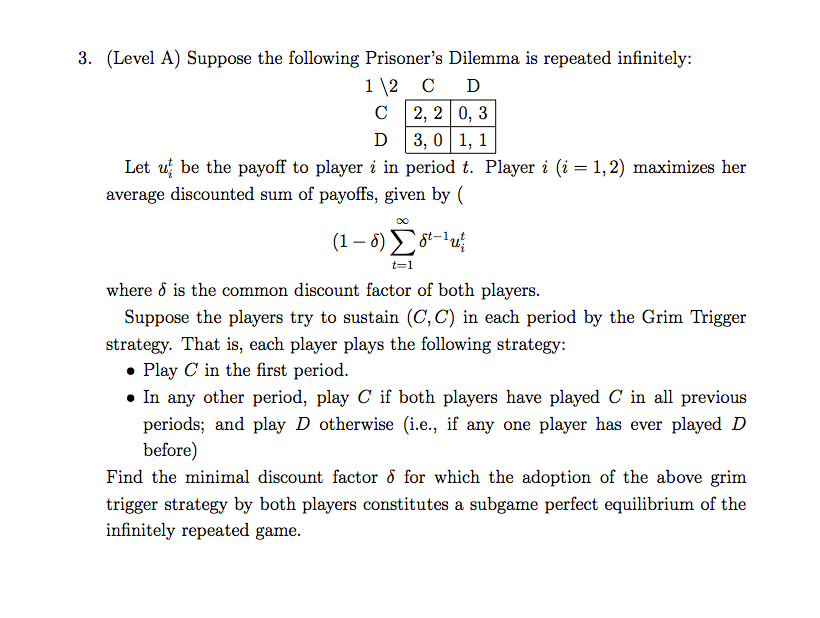3. (Level A) Suppose the following Prisoner's Dilemma is repeated infinitely: C 2, 2 0, 3 D 3,0 1, Let uj be the payoff to player i in period t. Player i (i 1,2) maximizes her average discounted sum of payoffs, given by ( o0 (1-6 X6u where o is the common discount factor of both players Suppose the players try to sustain (C, C) in each period by the Grim Trigger strategy. That is, each player plays the following...

• ### 3. (Level A) Suppose the following Prisoner's Dilemma is repeated infinitely 112 C D C 2,...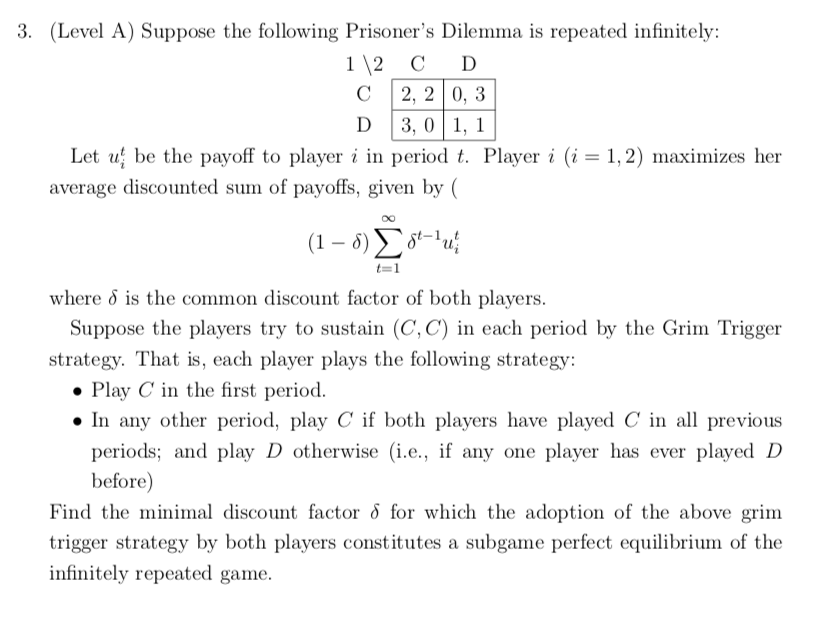3. (Level A) Suppose the following Prisoner's Dilemma is repeated infinitely 112 C D C 2, 2 0, 3 D 3, 0|1, 1 Let uļ be the payoff to player i in period t. Player i (i = 1, 2) maximizes her. average discounted sum of payoffs, given by ( where δ is the common discount factor of both players Suppose the players try to sustain (C, C) in each period by the Grim Trigger strategy. That is, each player...

• ### A game involving two players with two possible strategies is a prisoner's dilemma if each player...

A game involving two players with two possible strategies is a prisoner's dilemma if each player has a dominant strategy and: Select one: a. neither player plays their dominant strategy. b. each player's payoff is higher when both play their dominated strategy than when both play their dominant strategy. c. each player's payoff is lower when both play their dominant strategy than when both play their dominated strategy. d. there is a Nash equilibrium that yields the highest payoff for...

• ### 1. Consider the following normal form game: 112 L CR T 10 102 12 0 13...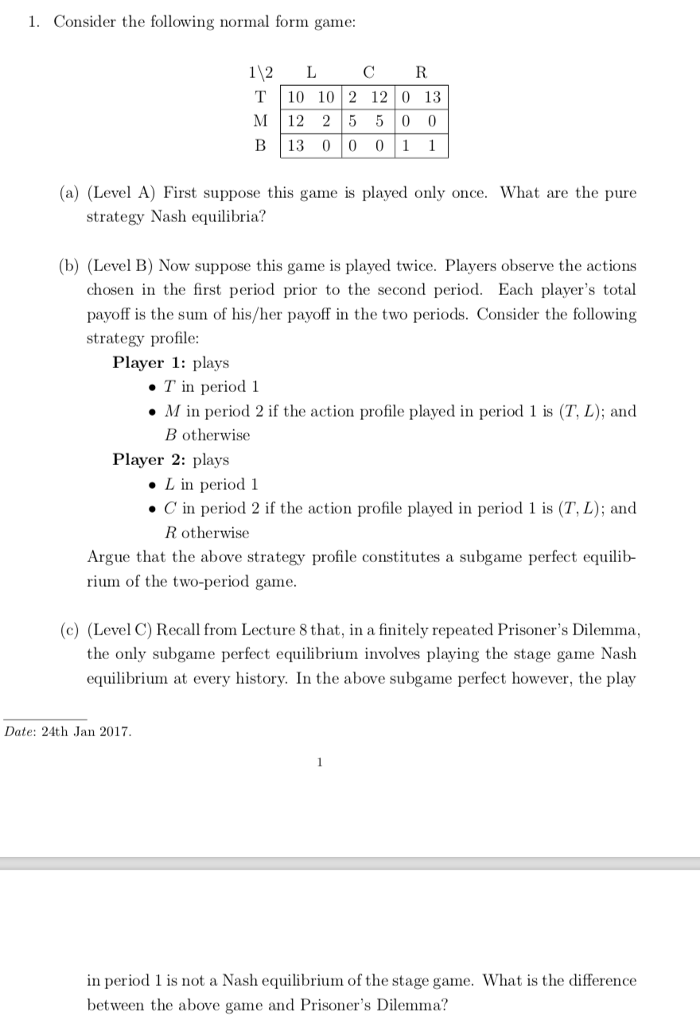1. Consider the following normal form game: 112 L CR T 10 102 12 0 13 M 12 25 5 0 0 B|13 010 011 a) (Level A) First suppose this game is played only once. What are the pure strategy Nash equilibria? (b) (Level B) Now suppose this game is played twice. Players observe the actions chosen in the first period prior to the second period. Each player's total payoff is the sum of his/her payoff in the two...

• ### 1. Consider the following normal form game 112 L CR T|10 1012 1210 13 M 12...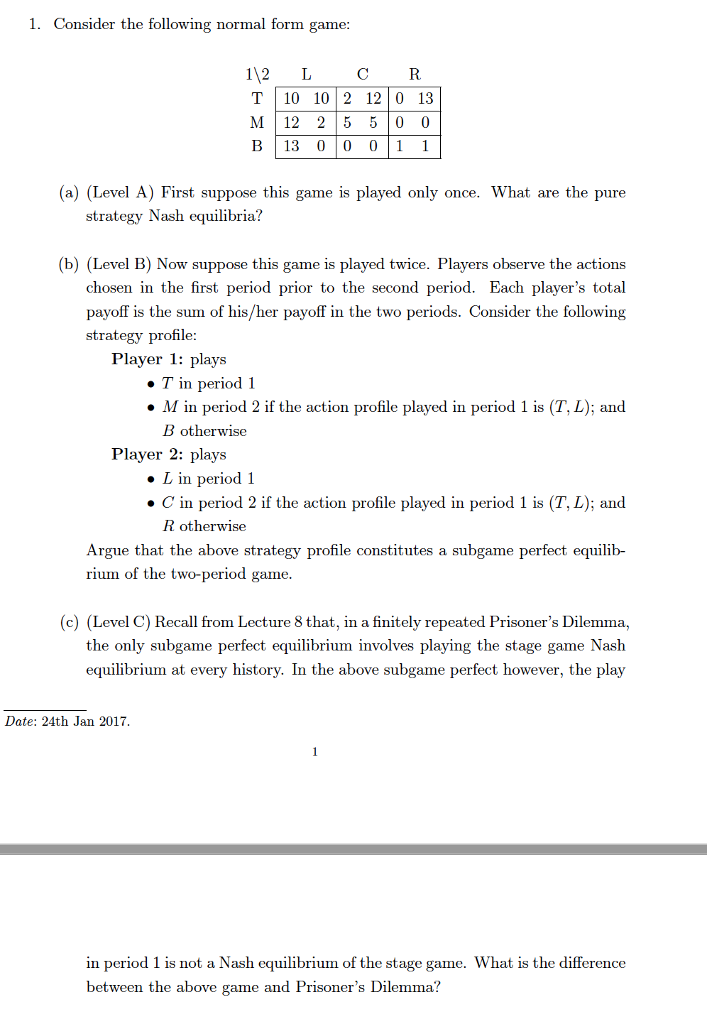1. Consider the following normal form game 112 L CR T|10 1012 1210 13 M 12 25 5 0 (0 B113 0100 (a) (Level A) First suppose this game is played only once. What are the pure strategy Nash equilibria? (b) (Level B) Now suppose this game is played twice. Players observe the actions chosen in the first period prior to the second period. Each player's total payoff is the sum of his/her payoff in the two periods. Consider the...

• ### You operate in a duopoly in which you and a rival must simultaneously decide what price...

You operate in a duopoly in which you and a rival must simultaneously decide what price to advertise in the weekly newspaper. If you each charge a low price, you each earn zero profits. If you each charge a high price, you each earn profits of \$3. If you charge different prices, the one charging the higher price loses \$5 and the one charging the lower price makes \$5. Your Rival Low Price High Price Low Price (0, 0) (5,...

• ### Below is a game matrix representing game a played between Congress, which is responsible for fiscal...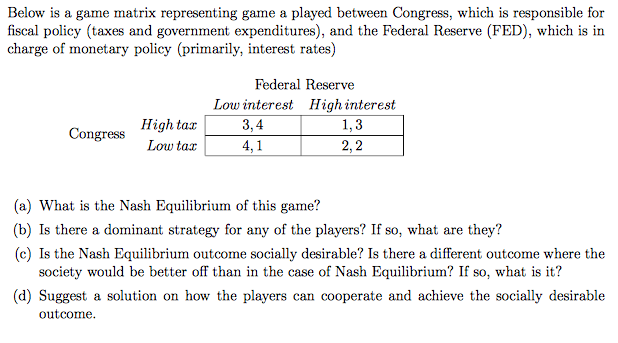Below is a game matrix representing game a played between Congress, which is responsible for fiscal policy (taxes and government expenditures), and the Federal Reserve (FED), which is in charge of monetary policy (primarily, interest rates) Federal Reserve Low interest Highintere:st Highta 3,4 Low tax 1,3 2,2 Congress (a) What is the Nash Equilibrium of this game? (b) Is there a dominant strategy for any of the playens? If so, what are they? (c) Is the Nash Equilibrium outcome socially...